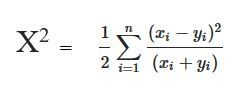# Chi-square distance in Python

Chi-square distance calculation is a statistical method, generally measures similarity between 2 feature matrices. Such distance is generally used in many applications like similar image retrieval, image texture, feature extractions etc. The Chi-square distance of 2 arrays ‘x’ and ‘y’ with ‘n’ dimension is mathematically calculated using below formula :In this article, we will learn how to calculate Chi-square distance using Python. Below given 2 different methods for calculating Chi-square Distance. Let’s see both of them with examples.

Method #1: Calculating Chi – square distance manually using above formula.

 `# importing numpy library ` `import` `numpy as np ` ` `  `# Function to calculate Chi-distace ` `def` `chi2_distance(A, B): ` ` `  `    ``# compute the chi-squared distance using above formula ` `    ``chi ``=` `0.5` `*` `np.``sum``([((a ``-` `b) ``*``*` `2``) ``/` `(a ``+` `b)  ` `                      ``for` `(a, b) ``in` `zip``(A, B)]) ` ` `  `    ``return` `chi ` ` `  `# main function ` `if` `__name__``=``=` `"__main__"``: ` `    ``a ``=` `[``1``, ``2``, ``13``, ``5``, ``45``, ``23``] ` `    ``b ``=` `[``67``, ``90``, ``18``, ``79``, ``24``, ``98``] ` ` `  `    ``result ``=` `chi2_distance(a, b) ` `    ``print``(``"The Chi-square distance is :"``, result) `

```Input : a = [1, 2, 13, 5, 45, 23]
b = [67, 90, 18, 79, 24, 98]
Output : The Chi-square distance is : 133.55428601494035

Input : a = [91, 900, 78, 30, 602, 813]
b = [57, 49, 36, 759, 234, 928]
Output :  The Chi-square distance is : 814.776999405035
```

Method #2: Using scipy.stats.chisquare() method

Syntax: scipy.stats.chisquare(f_obs, f_exp=None, ddof=0, axis=0)

Parameters:
==> f_obs : array1
==> f_exp : array2, optional
==> ddof(Delta degrees of freedom – adjustment for p-value) : int, optional
==> axis : int or None, optional
The default value of ddof and axis is 0.

Returns:
==> chisq : float or ndarray
==> p-value of the test : float or ndarray

 `# importing scipy ` `from` `scipy.stats ``import` `chisquare ` ` `  `k ``=` `[``3``, ``4``, ``6``, ``2``, ``9``, ``5``, ``2``] ` `print``(chisquare(k)) `

Output :

`Power_divergenceResult(statistic=8.516129032258064, pvalue=0.20267440425509237)`

My Personal Notes arrow_drop_upCheck out this Author's contributed articles.

If you like GeeksforGeeks and would like to contribute, you can also write an article using contribute.geeksforgeeks.org or mail your article to contribute@geeksforgeeks.org. See your article appearing on the GeeksforGeeks main page and help other Geeks.

Please Improve this article if you find anything incorrect by clicking on the "Improve Article" button below.

Article Tags :
Practice Tags :

Be the First to upvote.

Please write to us at contribute@geeksforgeeks.org to report any issue with the above content.